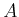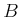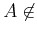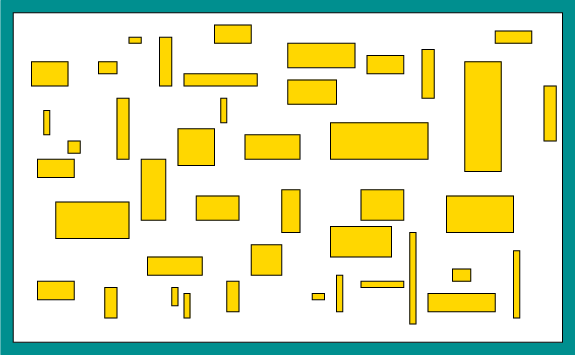#### Hardness and completeness

Since an easier class is included as a subset of a harder one, it is helpful to have a notion of a language (i.e., problem) being among the hardest possible within a class. Let X refer to either P, NP, PSPACE, or EXPTIME. A languageis called X-hard if every languagein class X is polynomial-time reducible to. In short, this means that in polynomial time, any language incan be translated into instances for language, and then the decisions forcan be correctly translated back in polynomial time to correctly decide. Thus, ifcan be decided, then within a polynomial-time factor, every language in X can be decided. The hardness concept can even be applied to a language (problem) that does not belong to the class. For example, we can declare that a languageis NP-hard even ifNP (it could be harder and lie in EXPTIME, for example). If it is known that the language is both hard for some class X and is also a member of X, then it is called X-complete (i.e., NP-complete, PSPACE-complete, etc.).6.8 Note that because of this uncertainty regarding P, NP, and PSPACE, one cannot say that a problem is intractable if it is NP-hard or PSPACE-hard, but one can, however, if the problem is EXPTIME-hard. One additional remark: it is useful to remember that PSPACE-hard implies NP-hard.Steven M LaValle 2020-08-14